## LeetCode刷題總結之雙指針法

1. 盛最多水的容器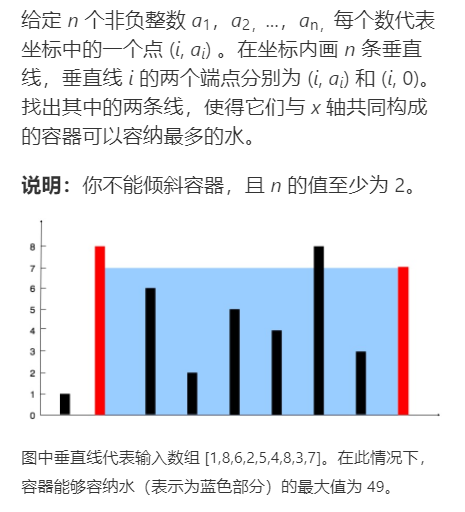int maxArea(vector<int>& height) {
int i=0,j=height.size()-1;
int maxA=0;
while(j-i>=1)
{
maxA=max(maxA,(min(height[i],height[j]))*(j-i));
if(height[i]<=height[j])
i++;
else
j--;
}
return maxA;
}
View Code

2. 三數之和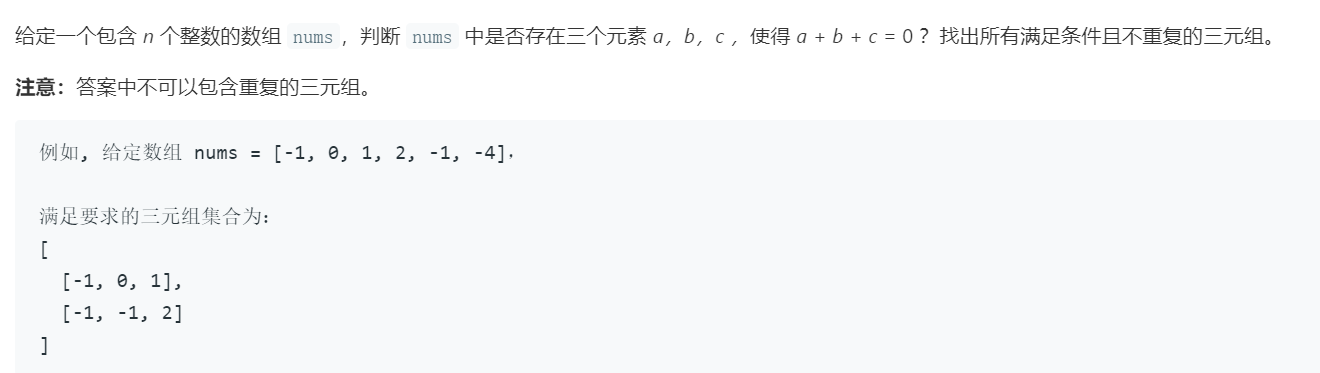vector<vector<int>> threeSum(vector<int>& nums) {
int n=nums.size();
sort(nums.begin(),nums.end());
if(n<3||nums>0||nums[n-1]<0)
return {};
vector<vector<int>> res;
for(int i=0;i<n-2;i++)
{
if(nums[i]>0)
break;
if(i>0&&nums[i]==nums[i-1])
continue;
int l=i+1,r=n-1;
while(l<r)
{
if(nums[l]+nums[r]==-nums[i])
{
vector<int> t;
t.push_back(nums[i]);
t.push_back(nums[l]);
t.push_back(nums[r]);
res.push_back(t);
l++;
r--;
while(l<r&&nums[l]==nums[l-1])
l++;
while(l<r&&nums[r]==nums[r+1])
r--;
}
else if(nums[l]+nums[r]<-nums[i])
l++;
else
r--;
}
}
return res;
}
View Code

3. 四數之和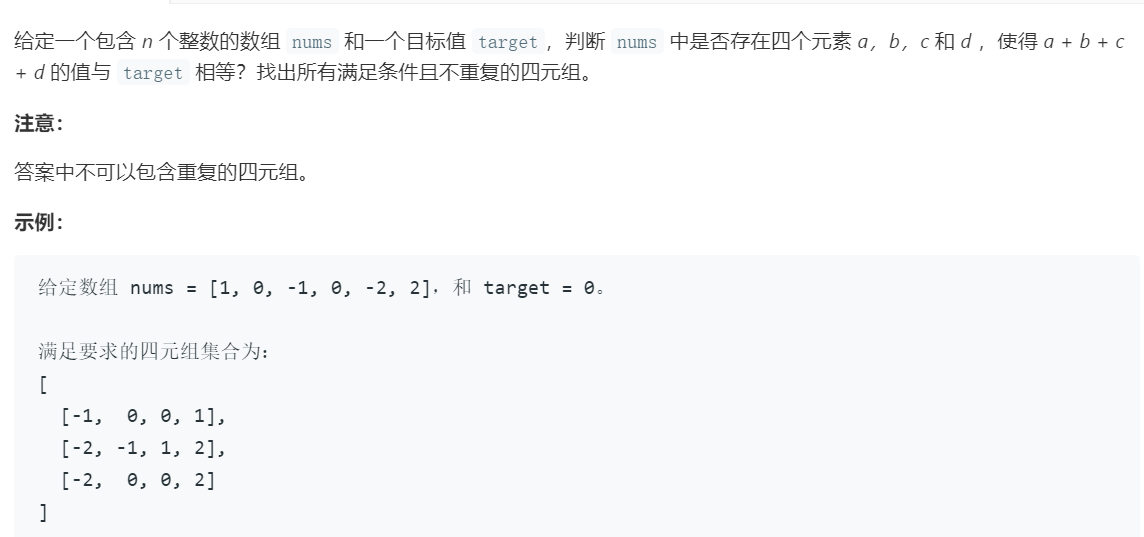、vector<vector<int>> fourSum(vector<int>& nums, int target) {
vector<vector<int>> res;
set<vector<int>> a;
int n=nums.size();
sort(nums.begin(),nums.end());
if(n<4)
return {};
for(int i=0;i<n-3;i++)
{
for(int j=i+1;j<n;j++)
{
int l=j+1,r=n-1;
while(l<r)
{
if(nums[i]+nums[j]+nums[l]+nums[r]==target)
{
a.insert(vector<int>{nums[i],nums[j],nums[l],nums[r],});
l++;
r--;
}
else if(nums[i]+nums[j]+nums[l]+nums[r]>target)
r--;
else
l++;
}
}
}
for(auto c:a)
{
res.push_back(c);
}
return res;
}
View Code

4. 最接近三數之和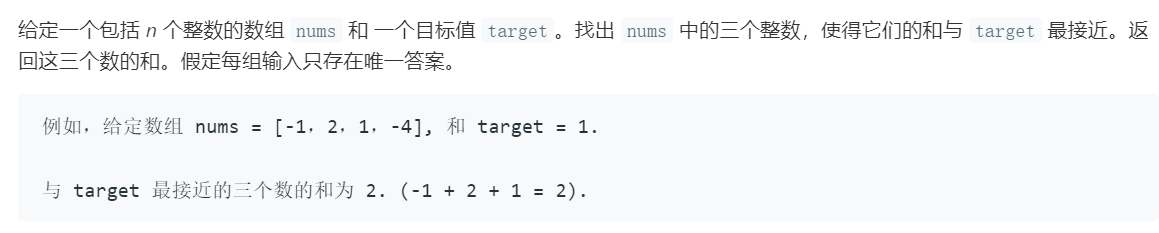int threeSumClosest(vector<int>& nums, int target) {
int n=nums.size();
sort(nums.begin(),nums.end());
int res;
int min=INT_MAX;
for(int i=0;i<n-2;i++)
{
int l=i+1,r=n-1;

while(l<r)
{
if(abs(target-nums[i]-nums[l]-nums[r])<min)
{
min=abs(target-nums[i]-nums[l]-nums[r]);
res=nums[i]+nums[l]+nums[r];
}
if(nums[i]+nums[l]+nums[r]>target)
r--;
else
l++;
}
}
return res;
}
View Code

5. 刪除鏈表的倒數第N個節點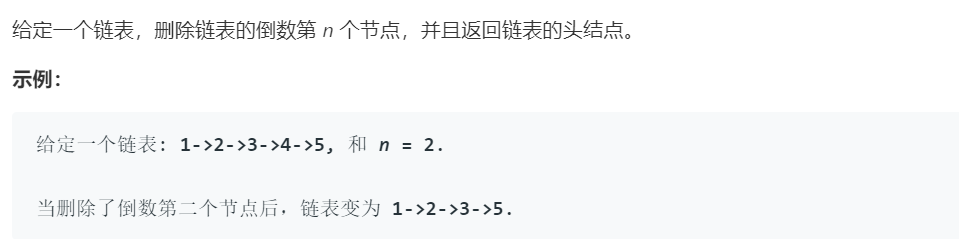ListNode* removeNthFromEnd(ListNode* head, int n) {

if(n==0)
for(int i=0;i<n;i++)
v=v->next;
if(v==NULL)
{
}
while(v->next!=NULL)
{
u=u->next;
v=v->next;
}
u->next=u->next->next;
}
View Code

6.  相交鏈表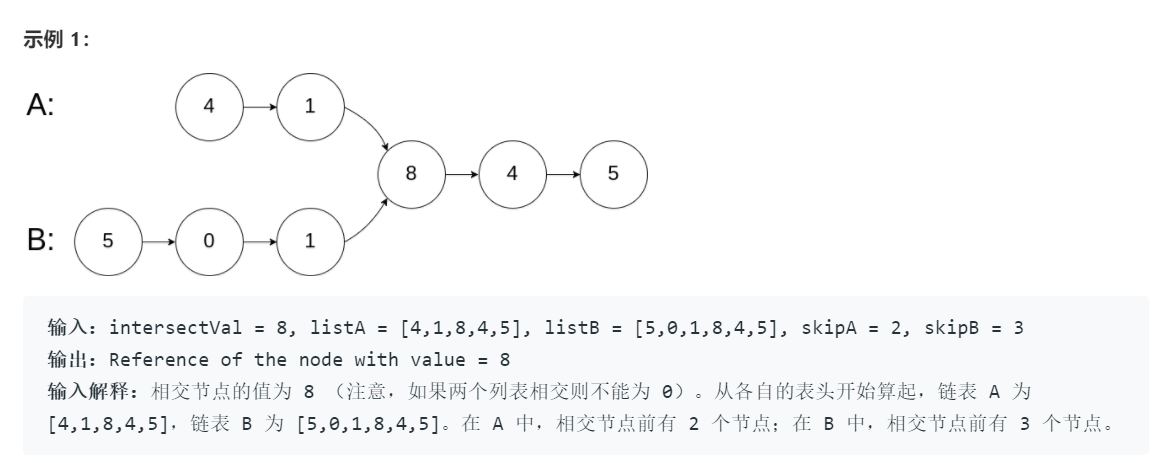• 創建兩個指針 pA
• 當 pApA 到達鏈表的尾部時，將它重定位到鏈表 B 的頭結點 (你沒看錯，就是鏈表 B); 類似的，當 pBpB 到達鏈表的尾部時，將它重定位到鏈表 A 的頭結點。

• 若在某一時刻 pAListNode *getIntersectionNode(ListNode *headA, ListNode *headB) {
int m=0,n=0;
while(p){
m++;
p=p->next;
}
while(q){
n++;
q=q->next;
}
if(m>n){
for(int i=0;i<m-n;i++)
p=p->next;
}
if(m<n){
for(int i=0;i<n-m;i++)
q=q->next;
}
while(p&&q&&p!=q){
p=p->next;
q=q->next;
}
if(!p) return NULL;
else return p;
}
View Code

7. 環形鏈表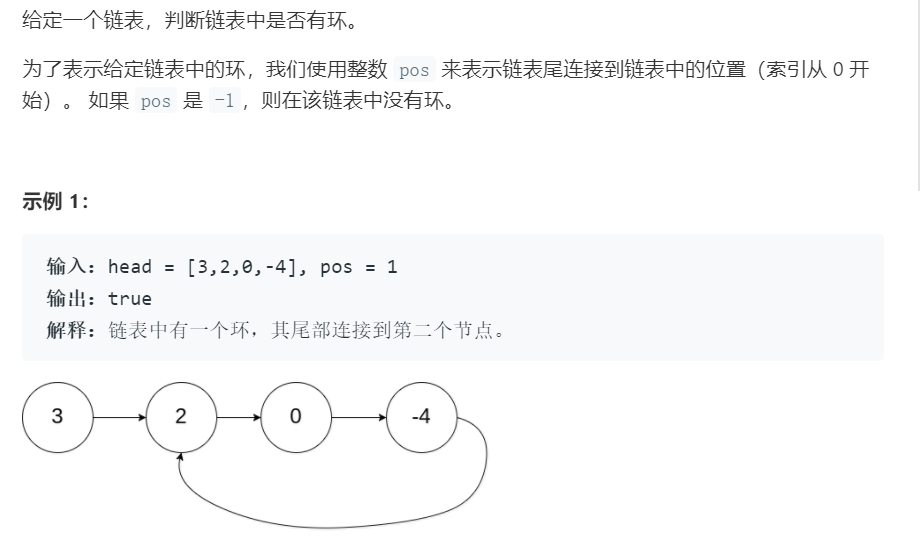bool hasCycle(ListNode *head) {
return 0;
while(s!=f)
{
if(f==NULL||f->next==NULL)
return 0;
s=s->next;
f=f->next->next;
}
return 1;
}
View Code

8. 回文鏈表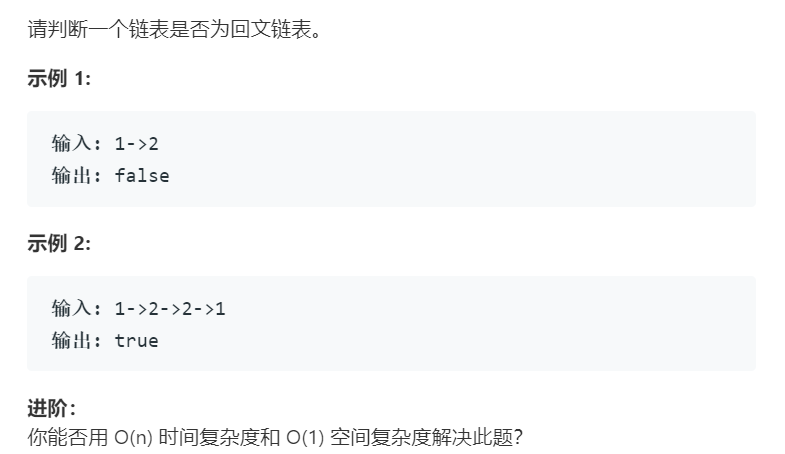1 bool isPalindrome(ListNode* head) {
3             return 1;
5         {
7                 return 1;
8             else
9                 return 0;
10         }
13         for(;fast&&fast->next;slow=slow->next,fast=fast->next->next)
14         ;
15         ListNode* back=reverseList(slow);
17             if(front->val!=back->val)
18                 return 0;
19         return 1;
20 }
22     {
25         ListNode* pre=NULL;
28         ListNode* res;
29         while(cur!=NULL)
30         {
31             if(next==NULL)
32                 res=cur;
33             else
34                 next=next->next;
35             cur->next=pre;
36             pre=cur;
37             cur=next;
38         }
39         return res;
40 }
View Code

posted on 2019-08-11 13:55  Mr..Prudence  閱讀(...)  評論(... 編輯 收藏